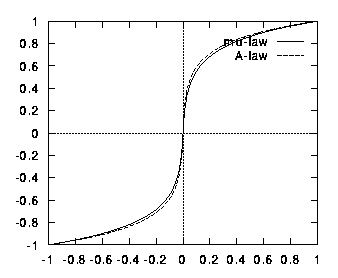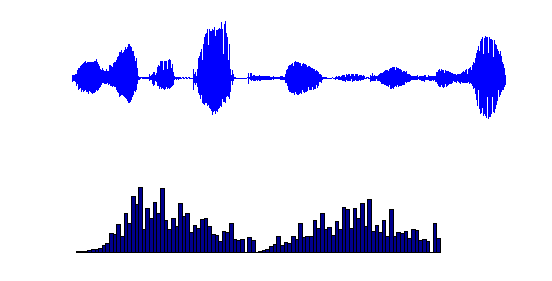Signal Quantization and Compression Overview

Sampling transforms a continuous-time signal into a discrete-time signal or sequence. The samples of the sequence can assume arbitrary values. However, in a digital implementation, real numbers have to be represented using a finite number of bits and the discrete-time sequence has therefore to be represented as a digital sequence. This can be achieved via quantization.

Quantization is a  nonlinear and irreversible operation that maps a given amplitude x(n) at time t=nT into a value xn, that belongs to a finite set of values.

### Linear Quantization

In this experiment we deal only with linear quantization where the finite set of values to choose from are uniformly spaced. More specifically, assume we are allowed only B bits to represent the amplitude of a sample. This means that for each sample, we should map its amplitude to only one of 2B possible levels. Now if the input signal is known to have a range (i.e., the amplitudes of its samples can only lie between -R /2 and +R /2),  then the spacing D between these levels is equal to D =R/2B.

Once we have identified or defined the finite set of  reconstruction levels, we can then choose a rule that allows us to map an amplitude to a certain reconstruction level. There are several ways to achieve this mapping and in this experiment we study three of them.

The optimal way to map from the input value to the reconstruction level is to round the input value to the closest reconstruction level. Another way  is to truncate an input value to the closest level that is smaller than it. A third way is to apply sign magnitude truncation, which maps an input value to the closest level whose absolute value is smaller than its absolute value.

Assume, for example, that the reconstruction levels are {-3,-1,1,3}. The amplitude 2.9 will be mapped to the level 3 if rounding is used, to the level 1 if truncation is used, and to the level 1 again if sign magnitude truncation is used. On the other hand, the amplitude -2.9 will be mapped to the level -3 if rounding is used, to the level -3 again if truncation is used, and to the level -1 if sign magnitude truncation is used.

### Companding Law

Narrowband speech is typically sampled 8000 times per second. Now assume we employ linear quantization to map the amplitudes of the samples to digital levels. It has been observed in practice that 12 bits per sample (i.e., B=12) are needed for very good quality speech, thus resulting in a bit rate of about 96 Kbits/s. We would like to lower the bit rate (since at this rate one minute of speech would require 6 MB of storage).

Assume the speech signal is normalized to the range R=1 (i.e., its samples lie between -1 and 1). If we examine a typical speech signal and its histogram, we shall see that we rarely use the extreme values +1 and -1 (see the figure below). Nonetheless, in a linear quantization scheme, we assign as many reconstruction levels for larger amplitudes as for smaller amplitudes, which are more probable to occur.A-law and u-law (pronounced mu-law) are so-called companding (COMPression - expANDING) schemes that are used in telephone networks (see figure below). They expand small values and compress large values. In other words, when a signal goes through a compander, small amplitudes are mapped into a larger interval and larger amplitudes are mapped into a smaller interval.  In this way, more quantization levels are used for the values that originated from small amplitudes. This scheme is  equivalent to applying non-uniform quantization to the original signal, where smaller quantization levels are used for smaller values and larger quantization levels are used for larger values.

.The above figures shows that  the range of input values (horizontal line) contained in the interval [-0.2,0.2] is mapped to the wider output interval [-0.6,0.6] (vertical line). On the other hand, the input intervals [-1,-0.6] and [0.6,1] are compressed to the outputs intervals [-0.9,-1] and [0.9,1]. The purpose of the mu-law companding function is therefore to equalize the histogram of the speech signal so that the reconstruction levels tend to be equally used.  The figure below shows the histogram of the same speech signal after mu-law companding.In this experiment, you can select among different sound signals and plot both the original signal and
its quantized version. You can also listen to both signals. The quantized signal that is shown is obtained
via sample-and-hold from the digital signal.